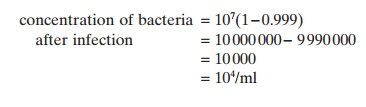Home | | Aquaculture Engineering | Basis of disinfection - Aquaculture Engineering

# Basis of disinfection - Aquaculture Engineering

Degree of removal, ChickŌĆÖs law, WatsonŌĆÖs law, Dose-response curve.

Basis of disinfection

## Degree of removal

The term percentage removal of actual micro-organisms is used in environmental engineering. In microbiological terms log10 removal or inactivation (decimal removal) is used to define the disinfection yield; normally a reduction of between 99 and 99.99% of the total number of bacteria is wanted, which corresponds to a log disinfection of 2ŌĆō4. However, these terms do not give exact values of the number of micro-organisms left; they only indicate by how much numbers are reduced from the starting concentration.

Example

The normal concentration of bacteria is 107/ml and a reduction of 99.9% is required. Find the concen-tration of bacteria present after disinfection.

Solution

concentration of bacteria after infection = 107(1 ŌłÆ 0.999)Example

The starting concentration of bacteria is 107/ml. A log disinfection of 3 is wanted. Calculate the new concentration of bacteria.

Solution

Let the starting concentration be N1 and the end con-centration N2; log(disinfection) = 3.

log(disinfection) = log(N1/N2)

logN1 ŌłÆ logN2log N2= log N1ŌłÆlog(disinfection)

=7 ŌĆō 3

=4

N2=104/ml

## ChickŌĆÖs law

Inactivation of micro-organisms in a disinfection plant depends on the time that the micro-organism are exposed to the disinfectant. This is described by ChickŌĆÖs law:

dn / dkN

where:

dn/dt= necessary time to inactivate n micro-organisms

=time constant depending on disinfectant,type of micro-organism and water quality

=number of live micro-organisms t =time.

This differential equation can be integrated within limits to give the following equation:

N1= N0eŌłÆkt

where:

N0=number of micro-organisms at the start

N1=number of micro-organisms after time t.

## WatsonŌĆÖs law

Based on the results of ChickŌĆÖs law, WatsonŌĆÖs law can be developed:where:

╬ø = coefficient of specific toxicity

=concentration of disinfectant

=exponent (normally around 1)

=time after start-up.

This means that the relation between the number of active and inactive micro-organisms is a product of the concentration of the disinfectant and the exposure time.

## Dose-response curve

Based on WatsonsŌĆÖs law a dose-response relation may be established for specific types of micro-organism. This gives the proportion of micro-organisms inactivated by fixed doses of disinfectant over various time periods. Exact dose-response relationships are difficult to determine in practice for several reasons. It is often difficult to isolate new pathogens and the response to a certain dose depends, amongst other factors, on the immune status of the organism, environmental conditions and population density.

Study Material, Lecturing Notes, Assignment, Reference, Wiki description explanation, brief detail
Aquaculture Engineering : Disinfection : Basis of disinfection - Aquaculture Engineering |